# 5.5. pyopus.problems.madsprob — Mesh adaptive direct search test problems¶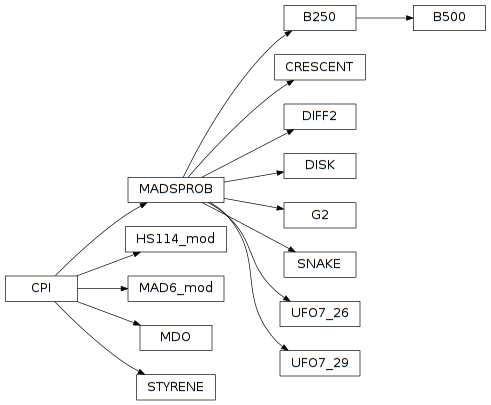Optimization test functions from papers on MADS. (PyOPUS subsystem name: MADSPROB)

All test functions in this module are maps from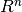to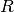.

The nc nonlinear constraints are of the form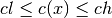Beside linear constraints a problem can also have bounds of the form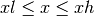This module is independent of PyOPUS, meaning that it can be taken as is and used as a module in some other package. It depends only on the cpi and the mgh module.

The STYRENE and the MDO problem depend on the _mads module. HS114 and MAD6 depend on the _lvns module.

References:

 [mads] Audet C., Dennis J.E. Jr., Mesh Adaptive Direct Search Algorithms for Constrained Optimization. SIAM Journal on Optimization, vol. 17, pp. 188-217, 2006.
 [madspsd] (1, 2, 3) Audet C., Dennis Jr J.E., Le Digabel S., Parallel Space Decomposition of the Mesh Adaptive Direct Search Algorithm. SIAM Journal on Optimization, vol. 19, pp. 1150-1170, 2008.
 [madsort] (1, 2) Abramson M.A., Audet C., Dennis J.E. Jr., Le Digabel S., ORTHO-MADS: A Deterministic MADS Instance with Orthogonal Directions. SIAM Journal on Optimization, vol. 20, pp. 948-966, 2009.
 [madspb] (1, 2, 3) Audet C., Dennis J.E. Jr., A Progressive Barrier for Derivative-Free Nonlinear Programming. SIAM Journal on Optimization, vol. 20, pp. 445-472, 2009.
 [madsqm] Conn A.R., Le Digabel S., Use of Quadratic Models with Mesh Adaptive Direct Search for Constrained Black Box Optimization. Optimization Methods and Software, vol. 28, pp. 139-158, 2013.
 [madsvns] (1, 2) Audet C., Bechard V., Le Digabel S., Nonsmooth Optimization Through Mesh Adaptive Direct Search and Variable Neighbourhood Search. Journal of Global Optimization, vol 41, pp. 299-318, 2008.
 [ufo] (1, 2) Lukšan L, et. al., UFO 2011 interactive system for univeral functional optimization. TR 1151 ICS, AS CR, 2011.

A list holding references to all function classes in this module.

SNAKE test function (n=2, nc=2).

Published as the first problem in section 4.1 of [madspb].

See the MADSPROB class for more details.

DISK test function (n=arbitrary, nc=1). Also referred to a the Hypersphere problem.

Published as the first problem in section 4.2 of [madspb] or as the first problem in section 5.3 of [mads].

See the MADSPROB class for more details.

CRESCENT test function (n=arbitrary, nc=2).

Published as the first problem in section 4.3 of [madspb].

See the MADSPROB class for more details.

G2 test function (n=arbitrary, nc=1).

Published as problem A in section 5.2 of [madspsd].

See the MADSPROB class for more details.

B250 test function (n=60, nc=1).

Mentioned as problem B in section 5.2 of [madspsd].

Rewritten from C++ source obtained from the NOMAD test problems collection.

See the MADSPROB class for more details.

B500 test function (n=60, nc=1).

Mentioned as problem B in section 5.2 of [madspsd].

Rewritten from C++ source obtained from the NOMAD test problems collection.

See the MADSPROB class for more details.

DIFF2 test function (n=2, nc=0).

Published as the problem (4.1) in [madsqm].

See the MADSPROB class for more details.

Problem from UFO manual (n, nc=int(3*(n-2)/2)).

Published as the first problem in section 7.26 of [ufo].

See the MADSPROB class for more details.

cpi()

Returns the common problem interface.

xmin is not available.

See the CPI class for more information.

Problem from UFO manual (n, nc=n-2).

Published as the first problem in section 7.29 of [ufo].

See the MADSPROB class for more details.

cpi()

Returns the common problem interface.

xmin is not available.

See the CPI class for more information.

Base class for the test functions

The fesible initial point can be obtained from the initial member. The infeasible initial point is in the initialinf member. If any of these two points is not given, it is set to None.

The full name of the problem is in the name member. The n member holds the dimension of the problem. nc is the number of nonlinear constraints.

The best known minimum value of the function is in the fmin member. If this value is unknown it is set to None.

Objects of this class are callable. The calling convention is

where x is the input values vector and gradients is a boolean flag specifying whether the gradients should be evaluated. The values of the auxiliary functions and their gradients are stored in the fi and J members. The values of the cobnstraints and the corresponding Jacobian are stored in the ci and J members.

This creates an instance of the CRESCENT function and evaluates the function and the constraints along with the gradient and the constraint Jacobian at the feasible initial point:

from pyopus.optimizer.madsprob import CRESCENT
cr=CRESCENT(n=10)
(f, g, c, Jc)=cr.fgcjc(cr.initial)

c(x)

Returns the value of the constraints at x.

cjc(x)

Returns the constraint Jacobian at x.

cpi()

Returns the common problem interface.

xmin is not available.

See the CPI class for more information.

f(x)

Returns the value of the test function at x.

fc(x)

Returns the value of teh function and constraints at x.

g(x)

Returns the value of the gradient at x.

name = None

The name of the test function

A multidisciplinary design optimization problem - maximization of aircraft range.

Warning - possible memory leaks and crashes the c++ code is not cleaned up.

cpi()

Returns the common problem interface.

xmin, f, and c are not available. Only fc is available.

See the CPI class for more information.

fc(x)

Returns the value of the function and the constraints at x.

An engineering optimization problem - styrene process optimization

Warning - possible memory leaks and crashes the c++ code is not cleaned up.

cpi()

Returns the common problem interface.

xmin, f, and c are not available. Only fc is available.

See the CPI class for more information.

fc(x)

Returns the value of the function and the constraints at x.

Modification of the MAD6 problem from the lvns module.

The MAD6 problem has 7 variables. One of them has an equality constraint imposed (x7). There is also a linear equality constraint involving x4 and x6. This modification eliminates x6 and x7 resulting in a problem with 5 variables and no equality constraint.

cpi()

Returns the common problem interface.

xmin, f, and c are not available. Only fc is available.

See the CPI class for more information.

fc(x)

Returns the value of the function and the constraints at x.

setup()

Initializes the binary implementation of the function. After this function is called no other function from the same test set may be created or initialized because that will change the internal variables and break the function. Returns an info structure.

Modification of the HS114 problem from the lvns module.

The HS114 problem has 10 variables. One linear equality constraint links x1, x4, and x5. This modification eliminates x5 resulting in a problem with 9 variables and no equality constraint.

cpi()

Returns the common problem interface.

xmin, f, and c are not available. Only fc is available.

See the CPI class for more information.

fc(x)

Returns the value of the function and the constraints at x.

setup()

Initializes the binary implementation of the function. After this function is called no other function from the same test set may be created or initialized because that will change the internal variables and break the function. Returns an info structure.

#### Previous topic

5.4. pyopus.problems.mwbm — More-Wild set of test functions for DFO, data profile generation

#### Next topic

5.6. pyopus.problems.lvns — Lukšan-Vlček set of nonsmooth problems